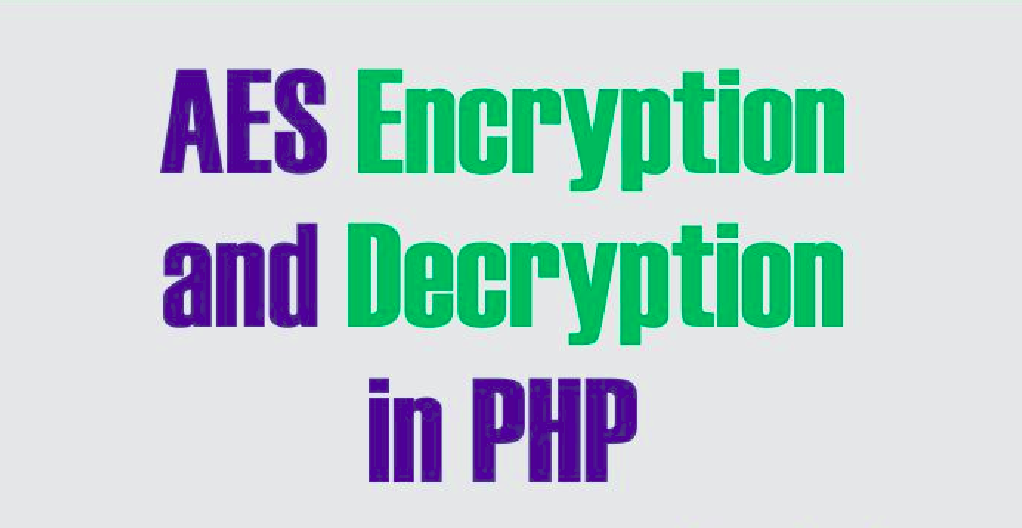# Basic AES-128-ECB Encryption/Decryption with PHPNote: ECB is useful for random data, but structured data should use a stronger mode like MCRYPT_MODE_CBC, Because ECB is an insecure method for sensitive data.

Lets see an example of its usage in PHP.

The Key must be 16bit long.

```    \$key = '1234567890123456';

function encrypt(\$data,\$key) {
return base64_encode(openssl_encrypt(\$data, "aes-128-ecb", \$key, OPENSSL_RAW_DATA));
}

function decrypt(\$data,\$key) {
return openssl_decrypt(base64_decode(\$data), "aes-128-ecb", \$key, OPENSSL_RAW_DATA);
}```

To Encrypt, Simply call

```\$data = "This is to be encrypted";
echo \$encrypted_text = encrypt(\$data,\$key);```

To Decrypt the above encrypted text

```\$data = "This is to be encrypted";
\$encrypted_text = encrypt(\$data,\$key);

\$data = \$encrypted_text;
echo \$decrypted_text = decrypt(\$data,\$key);```

But there are other problems in this code which make it insecure, in particular the use of ECB (which is not an encryption mode, only a building block on top of which encryption modes can be defined).

## Why ECB is insecure ?

You have a cipher, that with a key will encrypt 16 bytes of data. And you have some data, that is more than 16 bytes. Its a problem. ECB is the wrong solution to that problem: you just encrypt each 16-bytes block separately.

Why is it wrong? Because this way blocks that were equal before encryption will remain equal also after! And this will lead to all kinds of unwanted consequences.